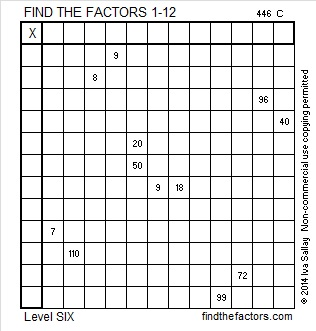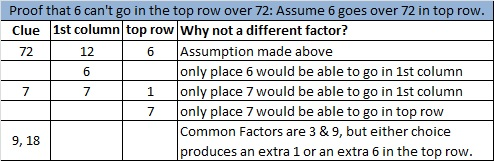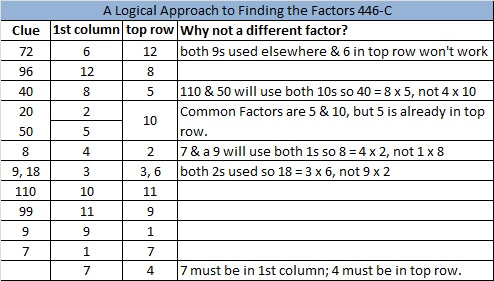302 and Level 6

• 302 is a composite number.
• Prime factorization: 302 = 2 x 151
• The exponents in the prime factorization are 1 and 1. Adding one to each and multiplying we get (1 + 1)(1 + 1) = 2 x 2 = 4. Therefore 302 has exactly 4 factors.
• Factors of 302: 1, 2, 151, 302
• Factor pairs: 302 = 1 x 302 or 2 x 151
• 302 has no square factors that allow its square root to be simplified. √302 ≈ 17.378Here’s a very tricky level 6 puzzle to try.Print the puzzles or type the factors on this excel file: 12 Factors 2014-11-17

The logic for this one is complicated. Here is an explanation that may be helpful:

9 is a clue two times in this puzzle. At most one of those 9’s can be 3 x 3. Clue 99 and the other nine will use both 9’s so 72 cannot be 8 x 9 in this puzzle. Here is an explanation of why 72 must be 6 x 12 with the 6 in the first column and the 12 in the top row.Now we can find the rest of the factors easily using logic: